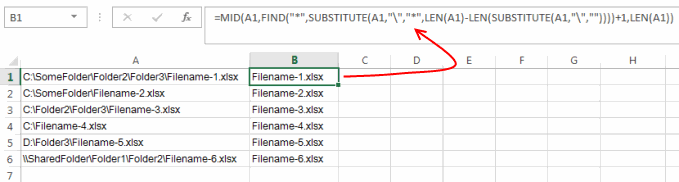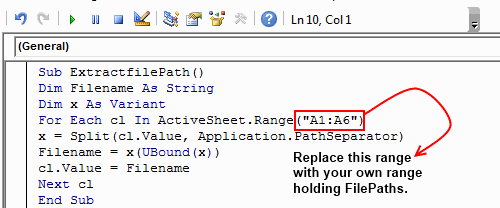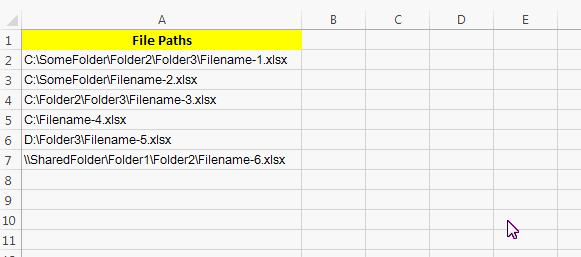# Extract Filenames from Filepath in Excel

Getting filenames from a Filepath looks an easy task at first sight. But actually this is a very cumbersome task when you are dealing with large number of file paths. Very recently I also wanted to do this task and during the process of extracting filenames I found few methods that can automate this process.

And today I will be sharing few methods that can help you to extract filenames from a given list of paths.Above picture can give you a clear idea of what I am actually talking about.

### Method 1: Fetch Filenames using a formula:

The first and one of the easiest ways to extract filename from file path is using a formula. Below formula can help you to do the same.

`=MID(A1,FIND("*",SUBSTITUTE(A1,"\","*",LEN(A1)-LEN(SUBSTITUTE(A1,"\",""))))+1,LEN(A1))`Here in this formula the only thing that you have to change is the cell position ‘A1’. While using this formula simply replace all the instances of cell position ‘A1’ with the cell position where you have file path present.

How this formula works:

I know many of you would be interested to know the logic behind the above formula. I will explain the above formula by breaking it into three parts:

1. First it finds how many “\” characters are present in the string. This is accomplished by `=LEN(A1)-LEN(SUBSTITUTE(A1,"\",""))` .

For instance your file path is “C:\SomeFolder\Folder\Filename.doc” then this part would give you a value “3”.

2. Secondly, it replaces the last “\” character of the input string by a “*” character. This is done by `=SUBSTITUTE(A1,"\","*",LEN(A1)-LEN(SUBSTITUTE(A1,"\","")))`

For example if your file path is “C:\SomeFolder\Folder\Filename.doc” then this part of the formula would give you a result “C:\SomeFolder\Folder*Filename.doc”

3. Now, your job becomes much easier. You only have to extract the part that follows “*” character. As you may be already knowing that this can be easily performed using the MID Function.

### Method 2: Using a Macro to extract File name from File path:

If you didn’t liked the above formula, then I have created a macro for you. This macro simply replaces the file paths in your predefined range with their corresponding File names. To use this macro follow the below steps:

1. With your excel sheet opened, Press the keys “Alt + F11”.

2. This will open the Excel VBA Editor, after this navigate to Insert > Module.

3. Now paste the below code in the editor.

`Sub ExtractfilePath()Dim Filename As StringDim x As VariantFor Each cl In ActiveSheet.Range("A1:A10")x = Split(cl.Value, Application.PathSeparator)Filename = x(UBound(x))cl.Value = FilenameNext clEnd Sub`4. Next, change the cell range (“A1:A10”). You have to change this range with your own range that contains file paths.

5. When you are ready to run the code simply press the “F5” key and all your file paths will be replaced by corresponding filenames.

### Method 3: By using Excel’s Find and Replace Functionality:

If you didn’t liked either of the two approaches that I have mentioned above then don’t worry here is a much easier alternative for you. This is done by using the Microsoft Excels in-built ‘Find and Replace’ functionality. Follow below steps to use this functionality to get the filenames from file paths.

1. First of all select the range where you have the FilePaths.

2. Next, press “Ctrl + H”, this will open a ‘Find and Replace’ dialog.3. In the “Find What” textbox enter “*\” (without quotes) and in keep the “Replace With” textbox empty. Click “Replace All” and all your Filepaths will be replaced by Filenames.

So, these were some of the methods for getting filenames from file paths. Do drop in your comments if you know any other methods.About Ankit Kaul

Ankit is the founder of Excel Trick. He is tech Geek who loves to sit in front of his square headed girlfriend (his PC) all day long. :D. Ankit has a strong passion for learning Microsoft Excel. His only aim is to turn you guys into 'Excel Geeks'.

1.Madarasays

Hello, Ankit!

While trying to solve my own problem with excel and looking throughout the www i stumbled on this article, and i have a hope, that you could help me with it.

So, here it goes:

I have this kind of excel filename: “Concrete element specification – Object name – Date.xls”
And the thing i want to achieve is that – in this specific file in a specific cell nr.1 automatically shows “Object name” and in another specific cell nr.2 shows “Date”.

Maybe You have an idea how to solve this problem? 🙂

Best regards,

•Ankit Kaulsays
`=TRIM(MID(MID(A1,FIND("–",A1)+1,100),1,FIND("–",MID(A1,FIND("–",A1)+1,100))-1)) `
`=TRIM(MID(MID(A1,FIND("–",A1)+1,100),FIND("–",MID(A1,FIND("–",A1)+1,100))+1,100))`